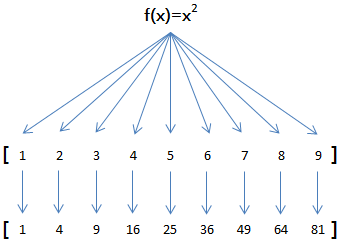# python中的map()函数和reduce()函数的用法

python内建了map()和reduce()函数。>>> def f(x):
… return x * x

>>> map(f, [1, 2, 3, 4, 5, 6, 7, 8, 9])
[1, 4, 9, 16, 25, 36, 49, 64, 81]

map()传入的第一个参数是f，即函数对象本身。

l = []
for n in [1, 2, 3, 4, 5, 6, 7, 8, 9]:
l.append(f(n))
print l

>>> map(str, [1, 2, 3, 4, 5, 6, 7, 8, 9])
[‘1’, ‘2’, ‘3’, ‘4’, ‘5’, ‘6’, ‘7’, ‘8’, ‘9’]

reduce(f, [x1, x2, x3, x4]) = f(f(f(x1, x2), x3), x4)

>>> def add(x, y):
… return x + y

>>> reduce(add, [1, 3, 5, 7, 9])
25

>>> def fn(x, y):
… return x * 10 + y

>>> reduce(fn, [1, 3, 5, 7, 9])
13579

>>> def fn(x, y):
… return x * 10 + y

>>> def char2num(s):
… return {‘0’: 0, ‘1’: 1, ‘2’: 2, ‘3’: 3, ‘4’: 4, ‘5’: 5, ‘6’: 6, ‘7’: 7, ‘8’: 8, ‘9’: 9}[s]

>>> reduce(fn, map(char2num, ‘13579’))
13579

def str2int(s):
def fn(x, y):
return x * 10 + y
def char2num(s):
return {‘0’: 0, ‘1’: 1, ‘2’: 2, ‘3’: 3, ‘4’: 4, ‘5’: 5, ‘6’: 6, ‘7’: 7, ‘8’: 8, ‘9’: 9}[s]
return reduce(fn, map(char2num, s))

def char2num(s):
return {‘0’: 0, ‘1’: 1, ‘2’: 2, ‘3’: 3, ‘4’: 4, ‘5’: 5, ‘6’: 6, ‘7’: 7, ‘8’: 8, ‘9’: 9}[s]

def str2int(s):
return reduce(lambda x,y: x*10+y, map(char2num, s))

python提供的sum()函数可以接受一个list并求和，请编写一个prod()函数，可以接受一个list并利用reduce()求积。

Posted in 未分类